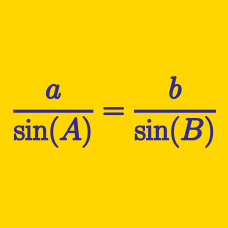Geometry

# Extended Sine Rule

In triangle $$ABC$$, let $$a, b$$ and $$c$$ be the lengths of the legs of a triangle opposite vertices $$A, B, C$$ respectively. Let $$\alpha, \beta, \gamma$$ be the values of the respective angles. What is the value of

$a ( \sin \beta - \sin \gamma) + b ( \sin \gamma - \sin \alpha ) + c ( \sin \alpha - \sin \beta )?$

Triangle $$ABC$$ is inscribed in a circle $$\Gamma$$. If $$\angle A = 60^{\circ}$$ and the length of the side opposite to vertex $$A$$ is $$49 \sqrt{3}$$, what is the radius of $$\Gamma$$?

In triangle $$ABC$$, $$\angle ABC = \frac{ \pi}{2}$$. If $$AC = 12$$, what is the radius of the circumcicle of $$ABC$$?

Triangle $$ABC$$ has $$\angle A=150^{\circ}$$ and $$\overline{BC}=7$$. If the area of the circumscribed circle of $$ABC$$ is $$a\pi$$, what is the value of $$a$$?

Triangle $$ABC$$ satisfies $$2\sin B=\sin A+\sin C$$. If $$a+c=30$$, what is the value of $$b$$?

Details and assumptions

$$a$$, $$b$$ and $$c$$ are the lengths of the sides opposite to the vertices $$A$$, $$B$$ and $$C$$, respectively.

×

Problem Loading...

Note Loading...

Set Loading...